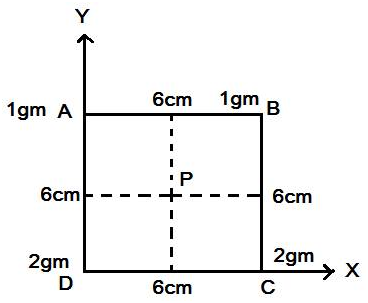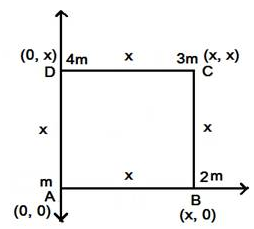# ROTATIONAL MOTION

• Home -
• ROTATIONAL MOTION

ROTATIONAL MOTION - Topic Wise Multiple Choice Questions (MCQ)

Topic Wise free Rotational Motion MCQ with solutions for 11th 12th exam Practice Multiple Choice Questions and answer for JEE-mains State CET Engineering Entrance GATE free Online Test

1 )

The centre of mass of systems of two particles is

on the line joining them and midway between them
on the line joining them at a point whose distance from each particle is proportional to the square of the mass of that particle.
on the line joining them at a point whose distance from each particle inversely proportional to the mass of that particle.
On the line joining them at a point whose distance from each particle is proportional to the mass of that particle.

View Solution

2 )

Particles of 1 gm, 1 gm, 2 gm, 2 gm are placed at the corners A, B, C, D, respectively of a square of side 6 cm as shown in figure. Find the distance of centre of mass of the system from geometrical centre of square.1 cm
2 cm
3 cm
4 cm

View Solution

3 )

Three particles of the same mass lie in the (X, Y) plane, The (X, Y) coordinates of their positions are (1, 1), (2, 2) and (3, 3) respectively. The (X, Y) coordinates of the centre of mass are

(1, 2)
(2, 2)
(1.5, 2)
(2, 1.5)

View Solution

4 )

Consider a two-particle system with the particles having masses M1, and M2. If the first particle is pushed towards the centre of mass through a distance d, by what distance should the second particle be moved so as to keep the centre of mass at the same position?

Select correct option from the following options

{A} [(M1d) / (M1 + M2)]          {B} [(M2d) / (M1 + M2)]

{C} [(M1d) / (M2)]                    {D} [(M2d) / (M1)]

A
B
C
D

View Solution

5 )

Four particles A, B, C and D of masses m, 2m, 3m and 4m respectively are placed at corners of a square of side x as shown in figure find the coordinate of centre of mass take A at origin of x-y plane.[2x, (7x / 10)]
[2x, (10x / 7)]
[(x / 2), (10x / 7)]
[(x / 2), (7x / 10)]

View Solution